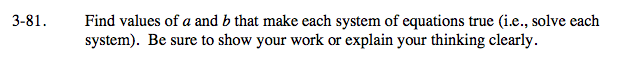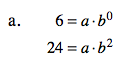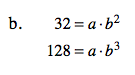### Home > CAAC > Chapter 14 > Lesson 14.3.1.6 > Problem3-81

3-81.Remember that b0 = 1.

You can use this fact to make the first equation 6 = a, and then substitute 6 for a in the second equation.

Solve for b.

24 = 6b2a = 2, b = 4